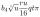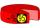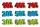# Cylinder twice

If the radius of the cylinder increases twice, and the height is reduced twice, then the volume of the cylinder increases (how many times?):

Result

n =  2

#### Solution:Leave us a comment of example and its solution (i.e. if it is still somewhat unclear...):

Showing 0 comments:Be the first to comment!#### To solve this example are needed these knowledge from mathematics:

Tip: Our volume units converter will help you with converion of volume units.

## Next similar examples:

1. BottleA company wants to produce a bottle whose capacity is 1.25 liters. Find the dimensions of a cylinder that will be required to produce this 1.25litres if the hight of the cylinder must be 5 times the radius.
2. A cylindrical tankA cylindrical tank can hold 44 cubic meters of water. If the radius of the tank is 3.5 meters, how high is the tank?
3. A pipeA radius of a cylindrical pipe is 2 ft. If the pipe is 17 ft long, what is its volume?
4. ConvaHow many liters of water fit into the shape of a cylinder with a bottom diameter 20 cm and a height 45 cm?
5. Rotary cylinder 2Base circumference of the rotary cylinder has same length as its height. What is the surface area of cylinder if its volume is 250 dm3?
6. Garden pondConcrete garden pond has bottom shape of a semicircle with a diameter 1.7 m and is 79 cm deep. Daddy wants make it surface. How many liters of water is in pond if watel level is 28 cm?
7. Common cylinderI've quite common example of a rotary cylinder. Known: S1 = 1 m2, r = 0.1 m Calculate : v =? V =? You can verify the results?
8. Total displacementCalculate total displacement of the 4-cylinder engine with the diameter of the piston bore B = 6.6 cm and stroke S=2.4 cm of the piston. Help: the crankshaft makes one revolution while the piston moves from the top of the cylinder to the bottom and back.
9. Circular poolThe 3.6-meter pool has a depth of 90 cm. How many liters of water is in the pool?
10. The potThe pot is a cylinder with a volume of V = 7l and an inner diameter of d = 20cm. Find its depth.
11. ContainerThe container has a cylindrical shape the base diameter 0.8 m and the area of the base is equal to the area of the wall. How many liters of water can we pour into the container?
12. Simplify 2Simplify expression: 5ab-7+3ba-9
13. ExpressionsExpression (3a-7).(3a+7) is identical with: ?
14. PercentagesExpressed as a percentage:
15. Greg and BillGreg is 18 years old. He is 6 less than 4 times Bill's age. How old is Bill?
16. Double percentWhat is 80% of 60% of 2800?
17. Simple equationsSolve system of equations: 5x+3y=5 5x+7y=25